# Selina Solutions Concise Maths Class 7 Chapter 10: Simple Interest

Selina Solutions Concise Maths Class 7 Chapter 10 Simple Interest contains problems on finding the interest in the given exercise problems. The main objective of providing solutions is to improve the analytical and problem solving abilities among students. For a better hold on the topics, students are advised to download Selina Solutions Concise Maths Class 7 Chapter 10 Simple Interest PDF, from the links given below.

Chapter 10 provides the basic idea about simple interest, principal, rate of interest, time period and amount. The method of determining them and the important formulas used in the process are explained in brief in the solutions PDF.

## Selina Solutions Concise Maths Class 7 Chapter 10: Simple Interest Download PDF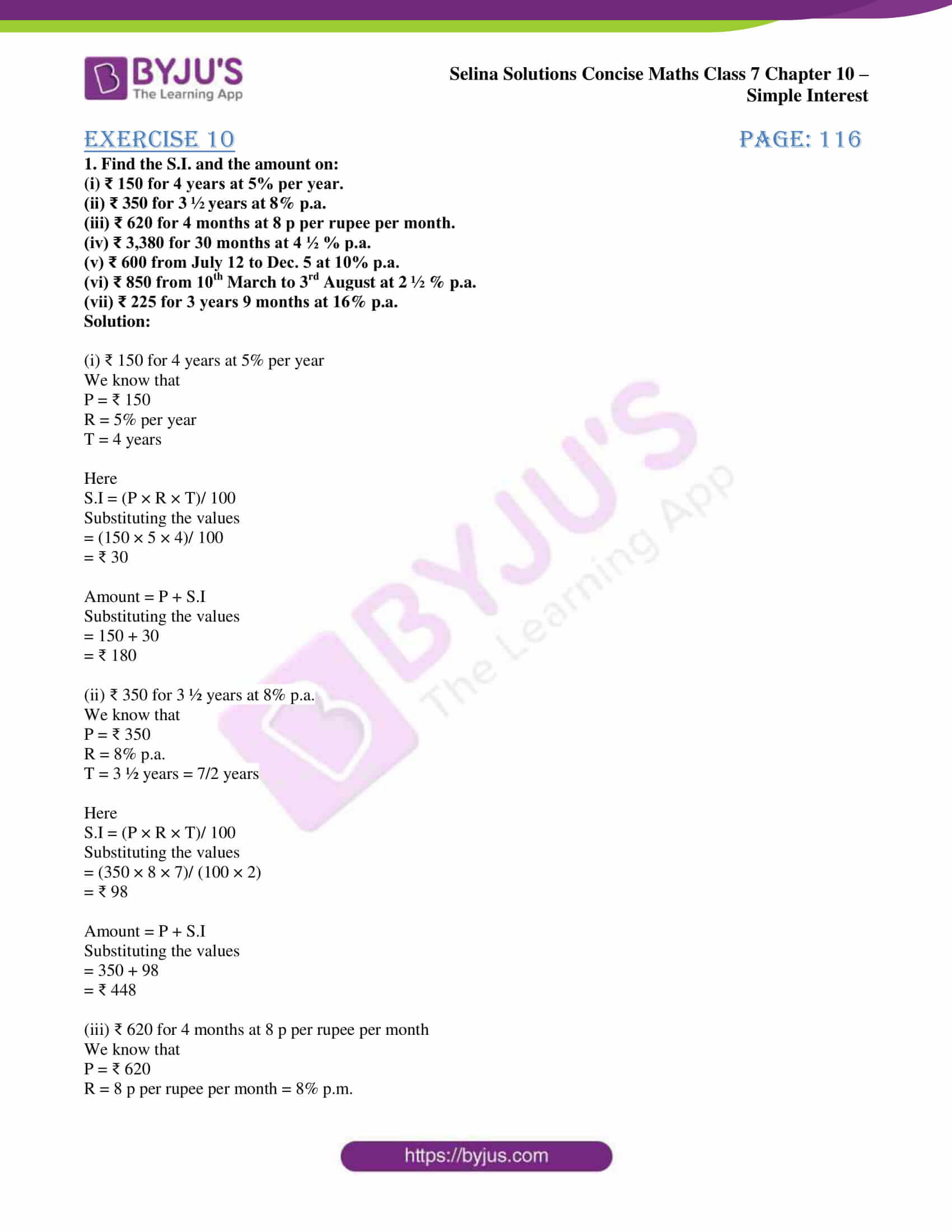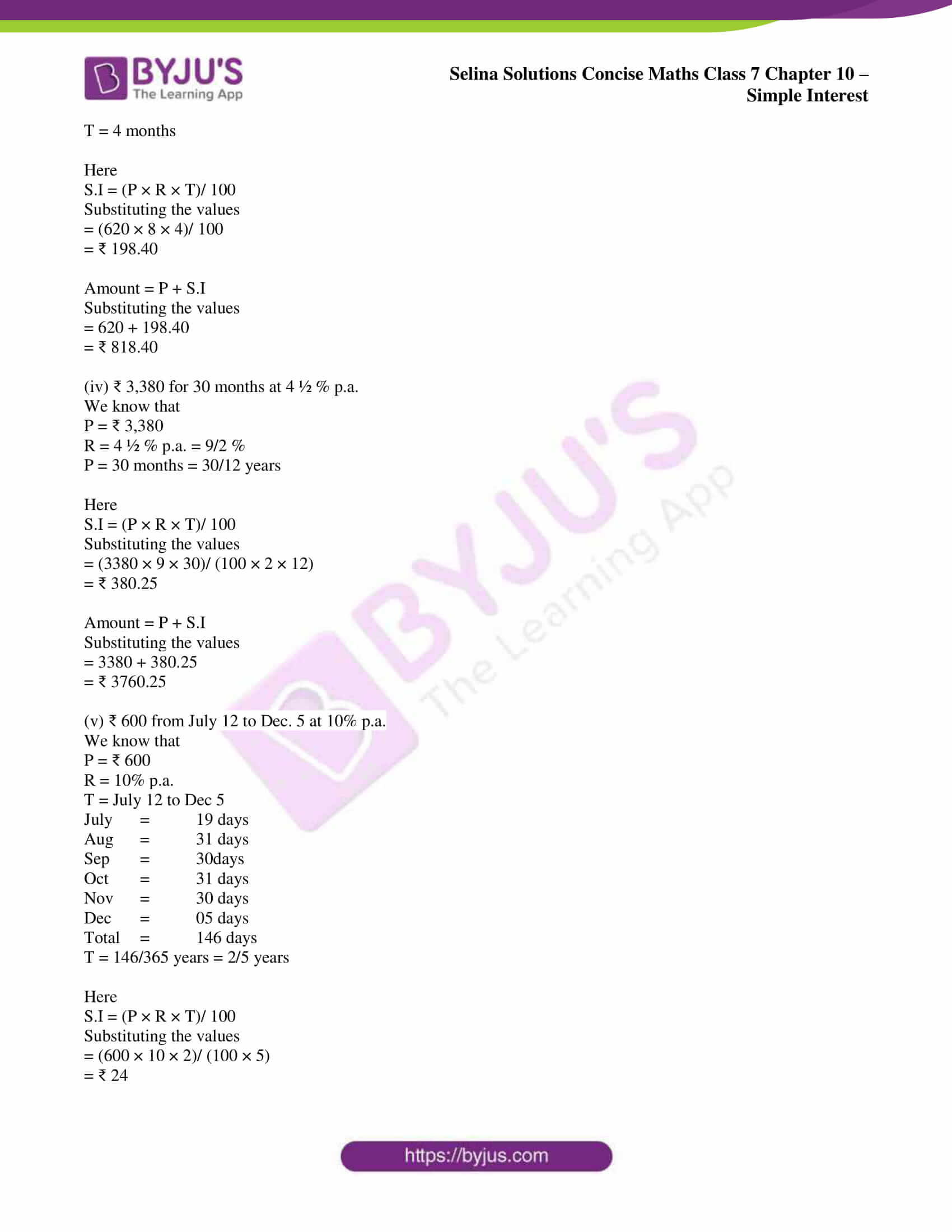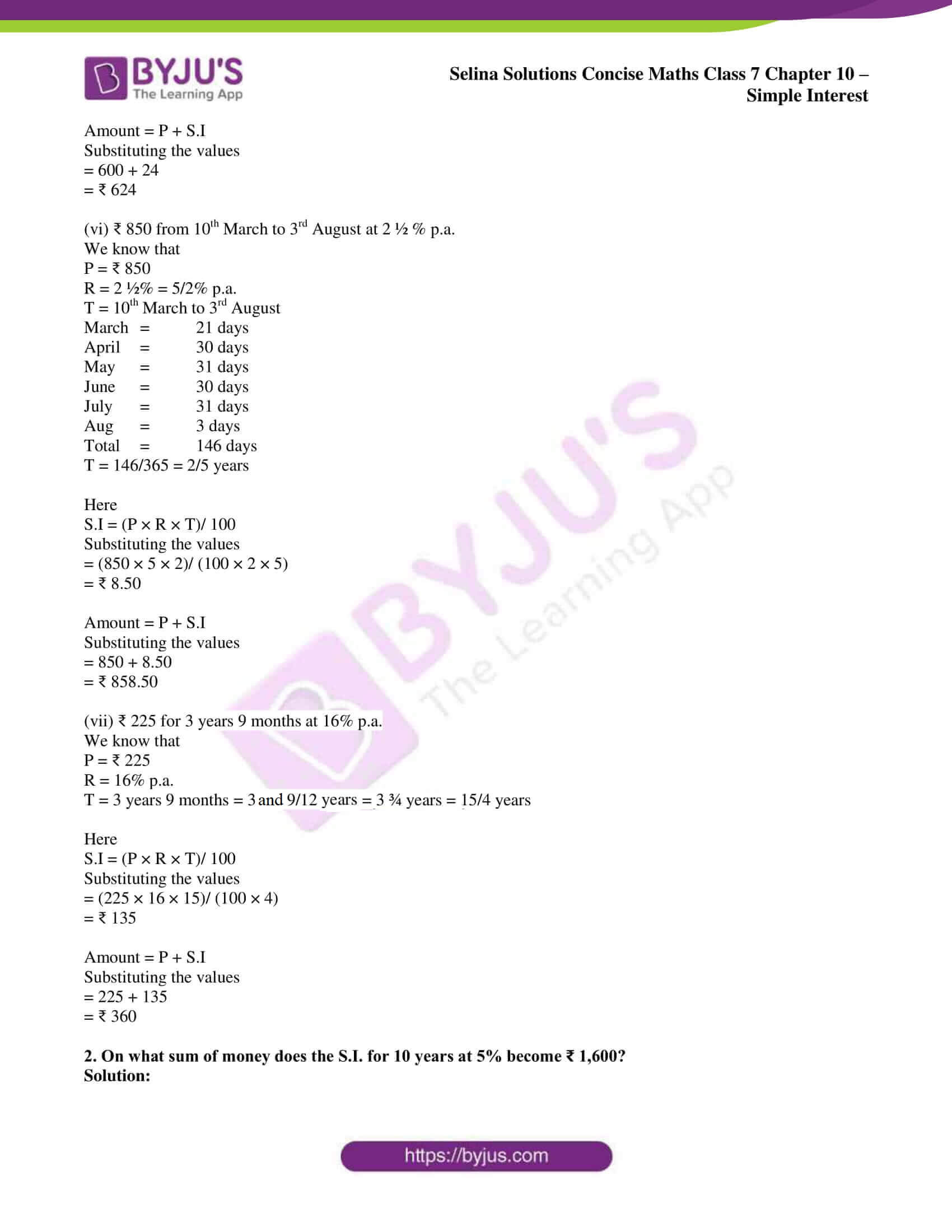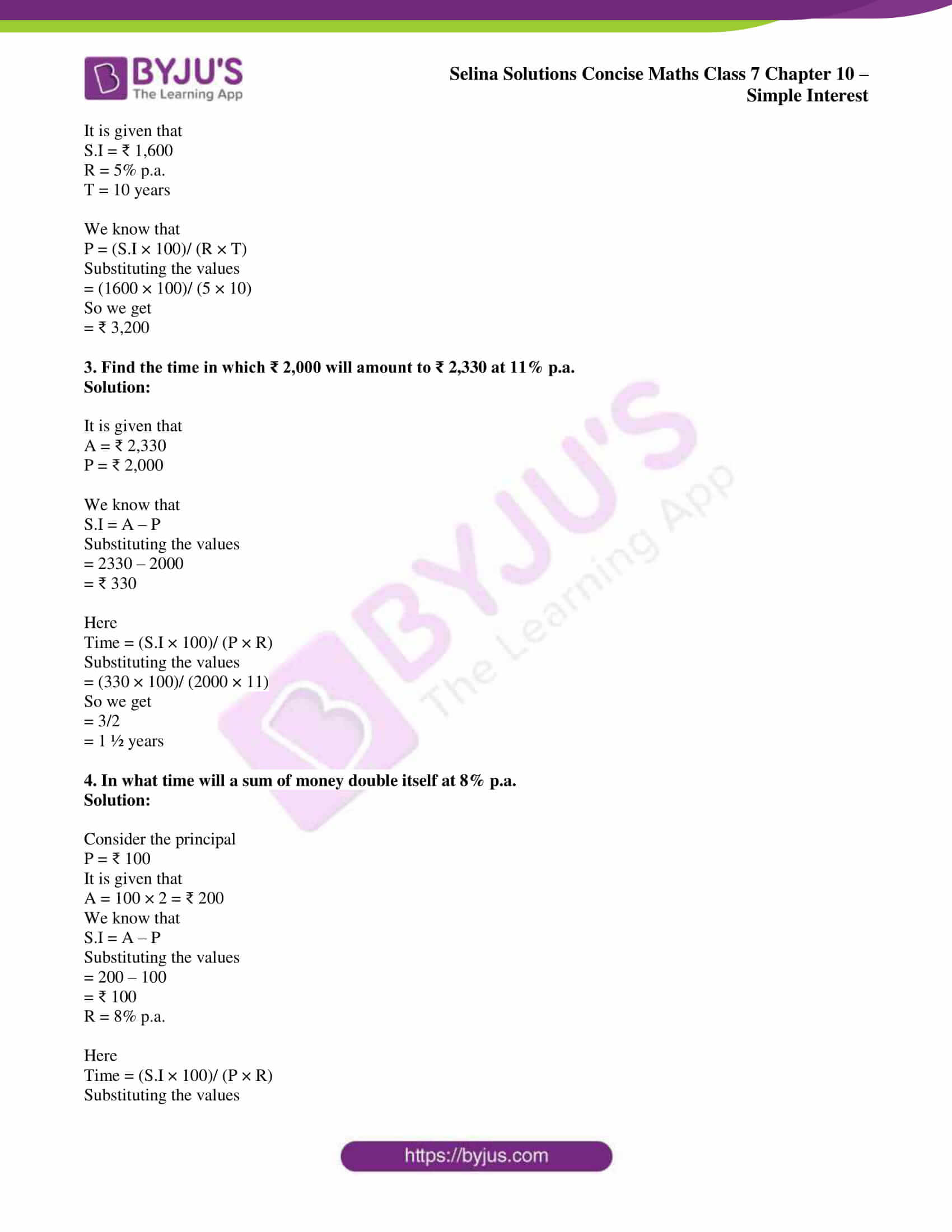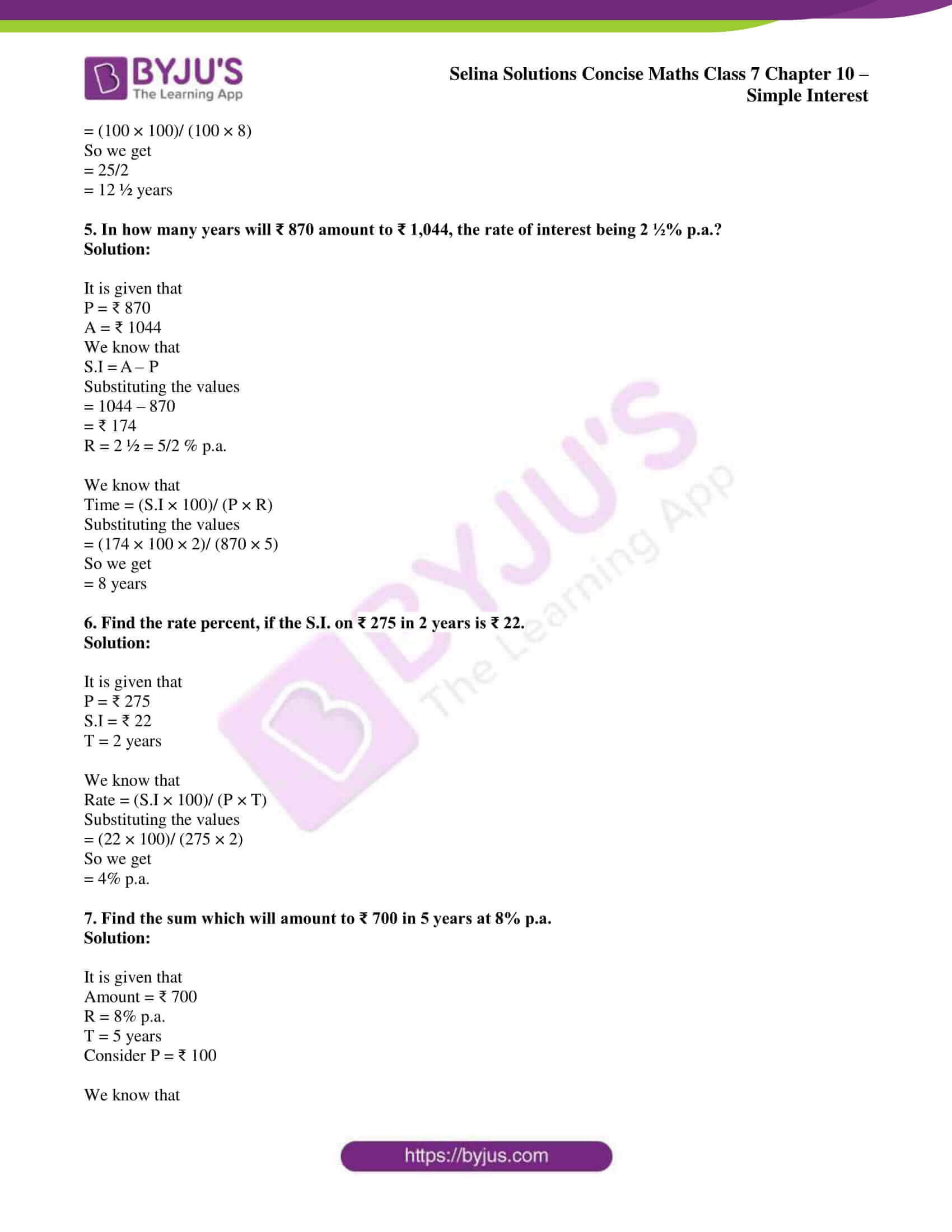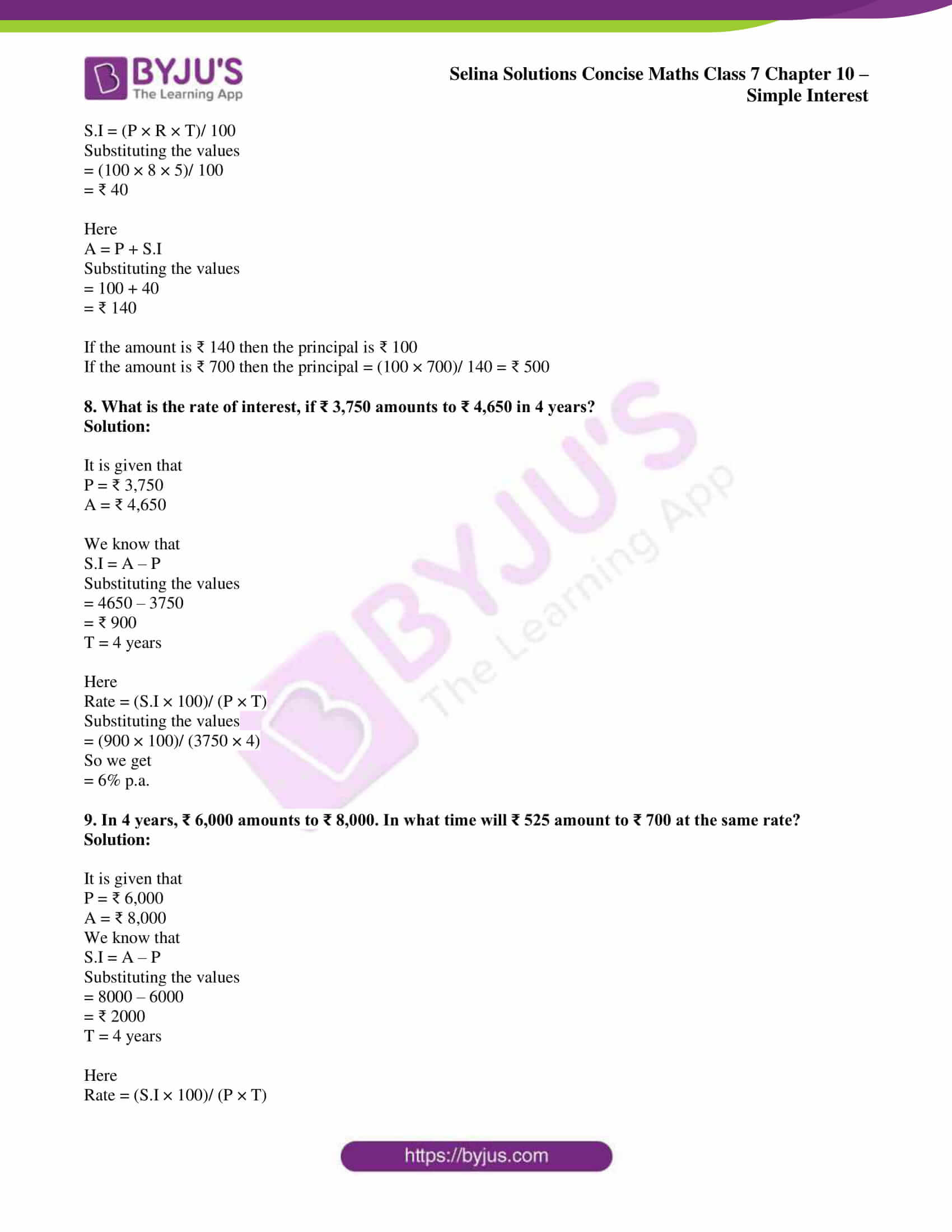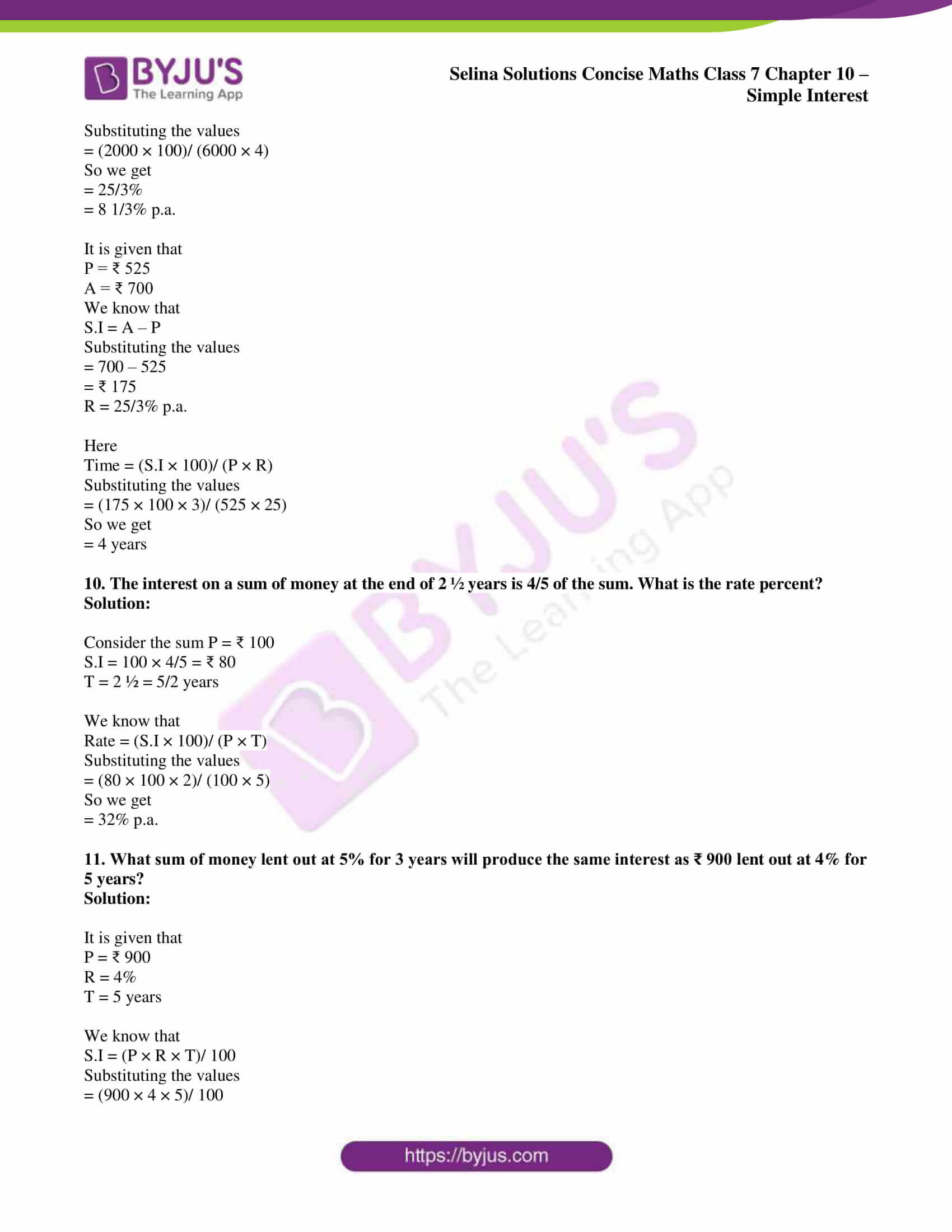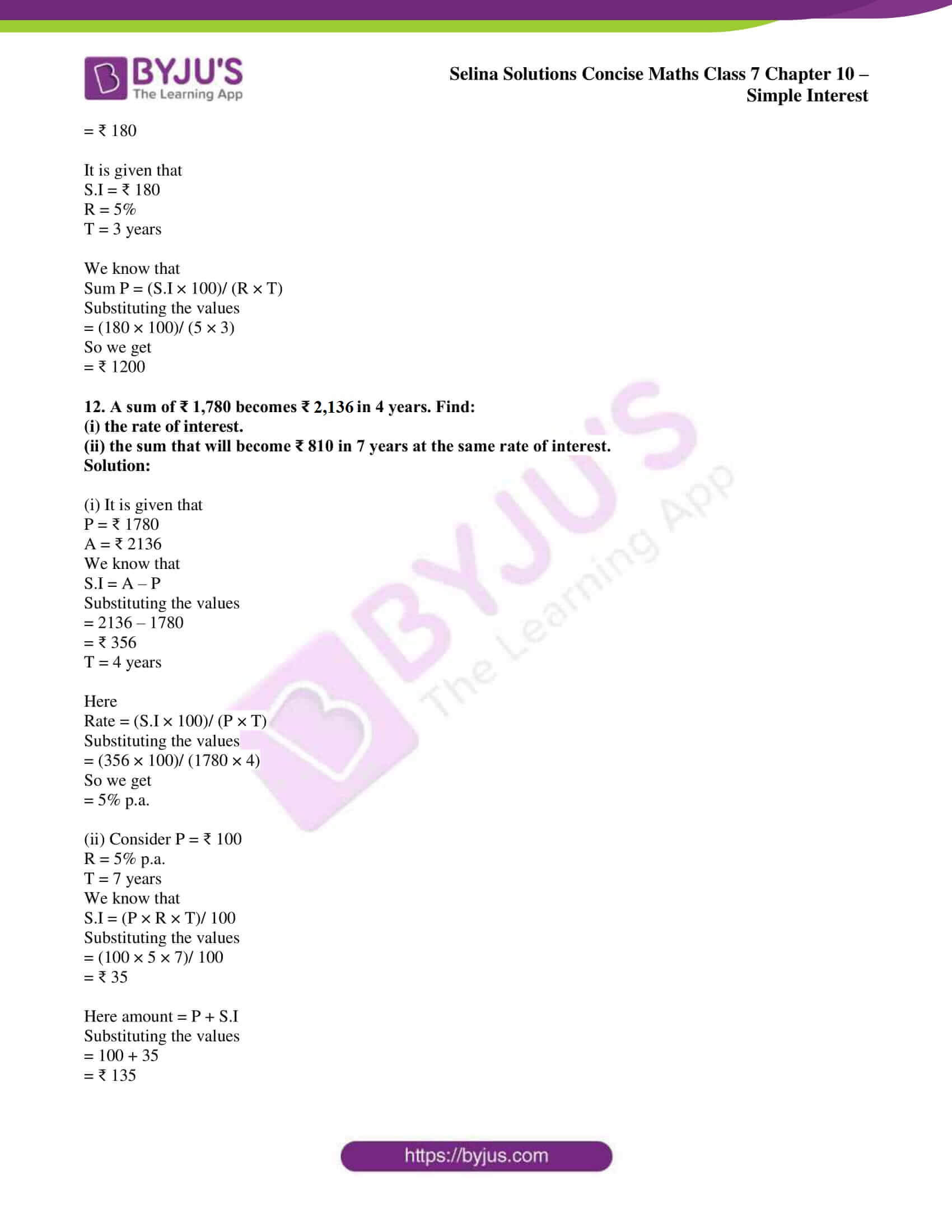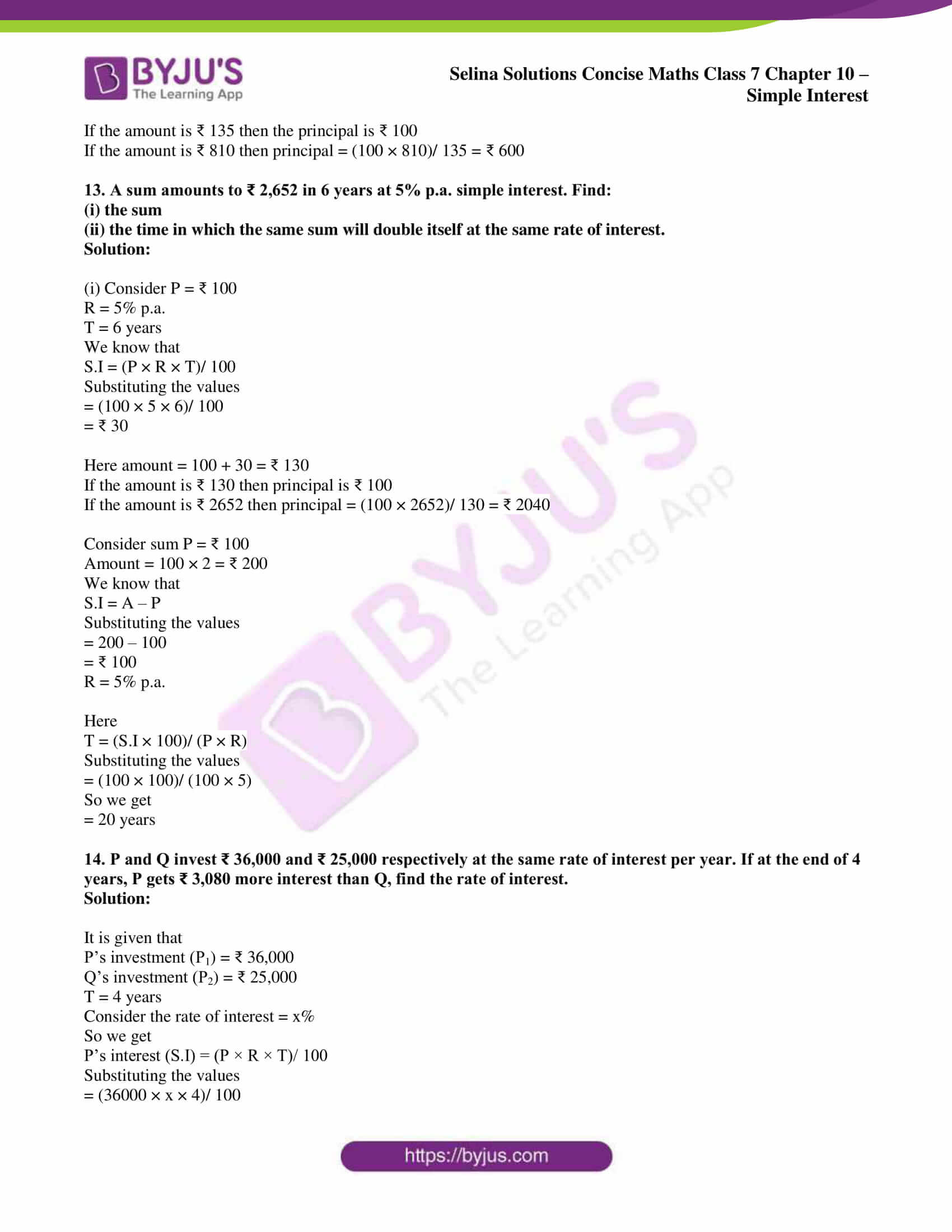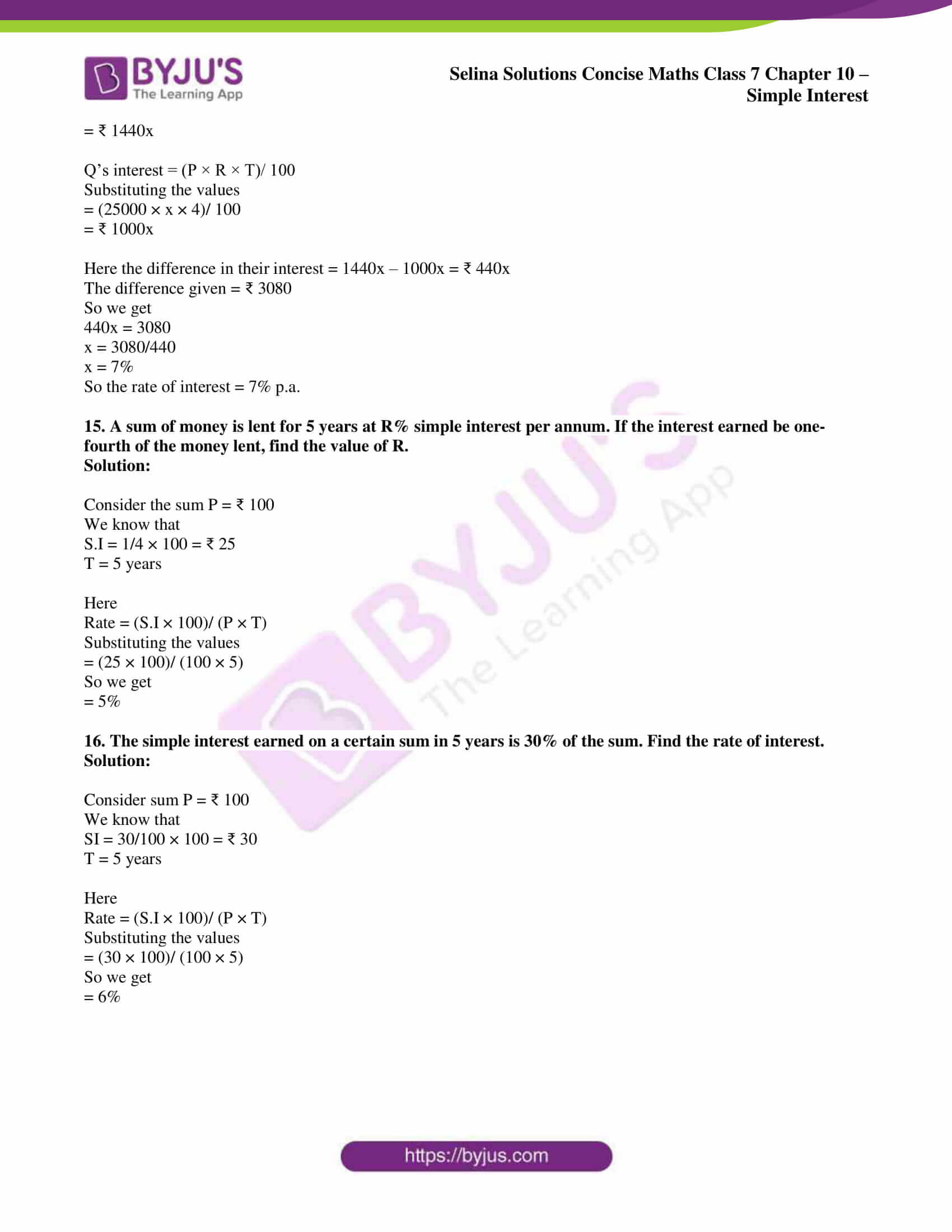## Access Selina Solutions Concise Maths Class 7 Chapter 10: Simple Interest

#### Exercise 10 page: 116

1. Find the S.I. and the amount on:

(i) ₹ 150 for 4 years at 5% per year.

(ii) ₹ 350 for 3 ½ years at 8% p.a.

(iii) ₹ 620 for 4 months at 8 p per rupee per month.

(iv) ₹ 3,380 for 30 months at 4 ½ % p.a.

(v) ₹ 600 from July 12 to Dec. 5 at 10% p.a.

(vi) ₹ 850 from 10th March to 3rd August at 2 ½ % p.a.

(vii) ₹ 225 for 3 years 9 months at 16% p.a.

Solution:

(i) ₹ 150 for 4 years at 5% per year

We know that

P = ₹ 150

R = 5% per year

T = 4 years

Here

S.I = (P × R × T)/ 100

Substituting the values

= (150 × 5 × 4)/ 100

= ₹ 30

Amount = P + S.I

Substituting the values

= 150 + 30

= ₹ 180

(ii) ₹ 350 for 3 ½ years at 8% p.a.

We know that

P = ₹ 350

R = 8% p.a.

T = 3 ½ years = 7/2 years

Here

S.I = (P × R × T)/ 100

Substituting the values

= (350 × 8 × 7)/ (100 × 2)

= ₹ 98

Amount = P + S.I

Substituting the values

= 350 + 98

= ₹ 448

(iii) ₹ 620 for 4 months at 8 p per rupee per month

We know that

P = ₹ 620

R = 8 p per rupee per month = 8% p.m.

T = 4 months

Here

S.I = (P × R × T)/ 100

Substituting the values

= (620 × 8 × 4)/ 100

= ₹ 198.40

Amount = P + S.I

Substituting the values

= 620 + 198.40

= ₹ 818.40

(iv) ₹ 3,380 for 30 months at 4 ½ % p.a.

We know that

P = ₹ 3,380

R = 4 ½ % p.a. = 9/2 %

P = 30 months = 30/12 years

Here

S.I = (P × R × T)/ 100

Substituting the values

= (3380 × 9 × 30)/ (100 × 2 × 12)

= ₹ 380.25

Amount = P + S.I

Substituting the values

= 3380 + 380.25

= ₹ 3760.25

(v) ₹ 600 from July 12 to Dec. 5 at 10% p.a.

We know that

P = ₹ 600

R = 10% p.a.

T = July 12 to Dec 5

July = 19 days

Aug = 31 days

Sep = 30days

Oct = 31 days

Nov = 30 days

Dec = 05 days

Total = 146 days

T = 146/365 years = 2/5 years

Here

S.I = (P × R × T)/ 100

Substituting the values

= (600 × 10 × 2)/ (100 × 5)

= ₹ 24

Amount = P + S.I

Substituting the values

= 600 + 24

= ₹ 624

(vi) ₹ 850 from 10th March to 3rd August at 2 ½ % p.a.

We know that

P = ₹ 850

R = 2 ½% = 5/2% p.a.

T = 10th March to 3rd August

March = 21 days

April = 30 days

May = 31 days

June = 30 days

July = 31 days

Aug = 3 days

Total = 146 days

T = 146/365 = 2/5 years

Here

S.I = (P × R × T)/ 100

Substituting the values

= (850 × 5 × 2)/ (100 × 2 × 5)

= ₹ 8.50

Amount = P + S.I

Substituting the values

= 850 + 8.50

= ₹ 858.50

(vii) ₹ 225 for 3 years 9 months at 16% p.a.

We know that

P = ₹ 225

R = 16% p.a.

T = 3 years 9 months = 3 and 9/12 years = 3 ¾ years = 15/4 years

Here

S.I = (P × R × T)/ 100

Substituting the values

= (225 × 16 × 15)/ (100 × 4)

= ₹ 135

Amount = P + S.I

Substituting the values

= 225 + 135

= ₹ 360

2. On what sum of money does the S.I. for 10 years at 5% become ₹ 1,600?

Solution:

It is given that

S.I = ₹ 1,600

R = 5% p.a.

T = 10 years

We know that

P = (S.I × 100)/ (R × T)

Substituting the values

= (1600 × 100)/ (5 × 10)

So we get

= ₹ 3,200

3. Find the time in which ₹ 2,000 will amount to ₹ 2,330 at 11% p.a.

Solution:

It is given that

A = ₹ 2,330

P = ₹ 2,000

We know that

S.I = A – P

Substituting the values

= 2330 – 2000

= ₹ 330

Here

Time = (S.I × 100)/ (P × R)

Substituting the values

= (330 × 100)/ (2000 × 11)

So we get

= 3/2

= 1 ½ years

4. In what time will a sum of money double itself at 8% p.a.

Solution:

Consider the principal

P = ₹ 100

It is given that

A = 100 × 2 = ₹ 200

We know that

S.I = A – P

Substituting the values

= 200 – 100

= ₹ 100

R = 8% p.a.

Here

Time = (S.I × 100)/ (P × R)

Substituting the values

= (100 × 100)/ (100 × 8)

So we get

= 25/2

= 12 ½ years

5. In how many years will ₹ 870 amount to ₹ 1,044, the rate of interest being 2 ½% p.a.?

Solution:

It is given that

P = ₹ 870

A = ₹ 1044

We know that

S.I = A – P

Substituting the values

= 1044 – 870

= ₹ 174

R = 2 ½ = 5/2 % p.a.

We know that

Time = (S.I × 100)/ (P × R)

Substituting the values

= (174 × 100 × 2)/ (870 × 5)

So we get

= 8 years

6. Find the rate percent, if the S.I. on ₹ 275 in 2 years is ₹ 22.

Solution:

It is given that

P = ₹ 275

S.I = ₹ 22

T = 2 years

We know that

Rate = (S.I × 100)/ (P × T)

Substituting the values

= (22 × 100)/ (275 × 2)

So we get

= 4% p.a.

7. Find the sum which will amount to ₹ 700 in 5 years at 8% p.a.

Solution:

It is given that

Amount = ₹ 700

R = 8% p.a.

T = 5 years

Consider P = ₹ 100

We know that

S.I = (P × R × T)/ 100

Substituting the values

= (100 × 8 × 5)/ 100

= ₹ 40

Here

A = P + S.I

Substituting the values

= 100 + 40

= ₹ 140

If the amount is ₹ 140 then the principal is ₹ 100

If the amount is ₹ 700 then the principal = (100 × 700)/ 140 = ₹ 500

8. What is the rate of interest, if ₹ 3,750 amounts to ₹ 4,650 in 4 years?

Solution:

It is given that

P = ₹ 3,750

A = ₹ 4,650

We know that

S.I = A – P

Substituting the values

= 4650 – 3750

= ₹ 900

T = 4 years

Here

Rate = (S.I × 100)/ (P × T)

Substituting the values

= (900 × 100)/ (3750 × 4)

So we get

= 6% p.a.

9. In 4 years, ₹ 6,000 amounts to ₹ 8,000. In what time will ₹ 525 amount to ₹ 700 at the same rate?

Solution:

It is given that

P = ₹ 6,000

A = ₹ 8,000

We know that

S.I = A – P

Substituting the values

= 8000 – 6000

= ₹ 2000

T = 4 years

Here

Rate = (S.I × 100)/ (P × T)

Substituting the values

= (2000 × 100)/ (6000 × 4)

So we get

= 25/3%

= 8 1/3% p.a.

It is given that

P = ₹ 525

A = ₹ 700

We know that

S.I = A – P

Substituting the values

= 700 – 525

= ₹ 175

R = 25/3% p.a.

Here

Time = (S.I × 100)/ (P × R)

Substituting the values

= (175 × 100 × 3)/ (525 × 25)

So we get

= 4 years

10. The interest on a sum of money at the end of 2 ½ years is 4/5 of the sum. What is the rate percent?

Solution:

Consider the sum P = ₹ 100

S.I = 100 × 4/5 = ₹ 80

T = 2 ½ = 5/2 years

We know that

Rate = (S.I × 100)/ (P × T)

Substituting the values

= (80 × 100 × 2)/ (100 × 5)

So we get

= 32% p.a.

11. What sum of money lent out at 5% for 3 years will produce the same interest as ₹ 900 lent out at 4% for 5 years?

Solution:

It is given that

P = ₹ 900

R = 4%

T = 5 years

We know that

S.I = (P × R × T)/ 100

Substituting the values

= (900 × 4 × 5)/ 100

= ₹ 180

It is given that

S.I = ₹ 180

R = 5%

T = 3 years

We know that

Sum P = (S.I × 100)/ (R × T)

Substituting the values

= (180 × 100)/ (5 × 3)

So we get

= ₹ 1200

12. A sum of ₹ 1,780 becomes ₹ 2,136 in 4 years. Find:

(i) the rate of interest.

(ii) the sum that will become ₹ 810 in 7 years at the same rate of interest.

Solution:

(i) It is given that

P = ₹ 1780

A = ₹ 2136

We know that

S.I = A – P

Substituting the values

= 2136 – 1780

= ₹ 356

T = 4 years

Here

Rate = (S.I × 100)/ (P × T)

Substituting the values

= (356 × 100)/ (1780 × 4)

So we get

= 5% p.a.

(ii) Consider P = ₹ 100

R = 5% p.a.

T = 7 years

We know that

S.I = (P × R × T)/ 100

Substituting the values

= (100 × 5 × 7)/ 100

= ₹ 35

Here amount = P + S.I

Substituting the values

= 100 + 35

= ₹ 135

If the amount is ₹ 135 then the principal is ₹ 100

If the amount is ₹ 810 then principal = (100 × 810)/ 135 = ₹ 600

13. A sum amounts to ₹ 2,652 in 6 years at 5% p.a. simple interest. Find:

(i) the sum

(ii) the time in which the same sum will double itself at the same rate of interest.

Solution:

(i) Consider P = ₹ 100

R = 5% p.a.

T = 6 years

We know that

S.I = (P × R × T)/ 100

Substituting the values

= (100 × 5 × 6)/ 100

= ₹ 30

Here amount = 100 + 30 = ₹ 130

If the amount is ₹ 130 then principal is ₹ 100

If the amount is ₹ 2652 then principal = (100 × 2652)/ 130 = ₹ 2040

Consider sum P = ₹ 100

Amount = 100 × 2 = ₹ 200

We know that

S.I = A – P

Substituting the values

= 200 – 100

= ₹ 100

R = 5% p.a.

Here

T = (S.I × 100)/ (P × R)

Substituting the values

= (100 × 100)/ (100 × 5)

So we get

= 20 years

14. P and Q invest ₹ 36,000 and ₹ 25,000 respectively at the same rate of interest per year. If at the end of 4 years, P gets ₹ 3,080 more interest than Q, find the rate of interest.

Solution:

It is given that

P’s investment (P1) = ₹ 36,000

Q’s investment (P2) = ₹ 25,000

T = 4 years

Consider the rate of interest = x%

So we get

P’s interest (S.I) = (P × R × T)/ 100

Substituting the values

= (36000 × x × 4)/ 100

= ₹ 1440x

Q’s interest = (P × R × T)/ 100

Substituting the values

= (25000 × x × 4)/ 100

= ₹ 1000x

Here the difference in their interest = 1440x – 1000x = ₹ 440x

The difference given = ₹ 3080

So we get

440x = 3080

x = 3080/440

x = 7%

So the rate of interest = 7% p.a.

15. A sum of money is lent for 5 years at R% simple interest per annum. If the interest earned be one-fourth of the money lent, find the value of R.

Solution:

Consider the sum P = ₹ 100

We know that

S.I = 1/4 × 100 = ₹ 25

T = 5 years

Here

Rate = (S.I × 100)/ (P × T)

Substituting the values

= (25 × 100)/ (100 × 5)

So we get

= 5%

16. The simple interest earned on a certain sum in 5 years is 30% of the sum. Find the rate of interest.

Solution:

Consider sum P = ₹ 100

We know that

SI = 30/100 × 100 = ₹ 30

T = 5 years

Here

Rate = (S.I × 100)/ (P × T)

Substituting the values

= (30 × 100)/ (100 × 5)

So we get

= 6%